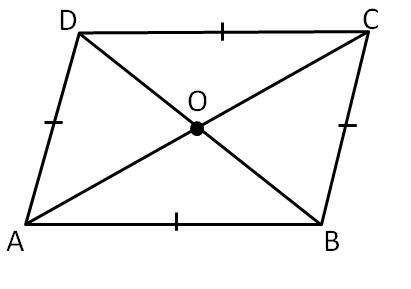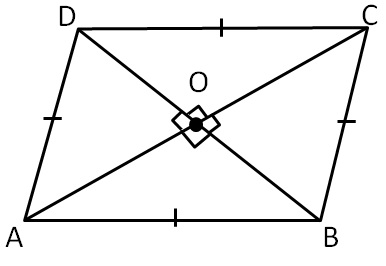Rhombus, Rectangle, Square

Chapter 3 Class 8 Understanding Quadrilaterals
Concept wiseRhombus is a parallelogram where

all sides are equal

i.e. AB = BC = CD = AD

In parallelogram

• Opposite sides are equal
• Opposite angles are equal
• Diagonals bisect each other
• Sum of adjacent angles is 180°

Properties of Rhombus:

Since it is a parallelogram,

it will have all its propertiesOther properties are

Diagonals bisect each other at right angles

AO = CO and BO = DO

and

∠ AOB = 90°

∠ BOC = 90°

∠ COD = 90°

∠ AOD = 90°

Learn in your speed, with individual attention - Teachoo Maths 1-on-1 Class